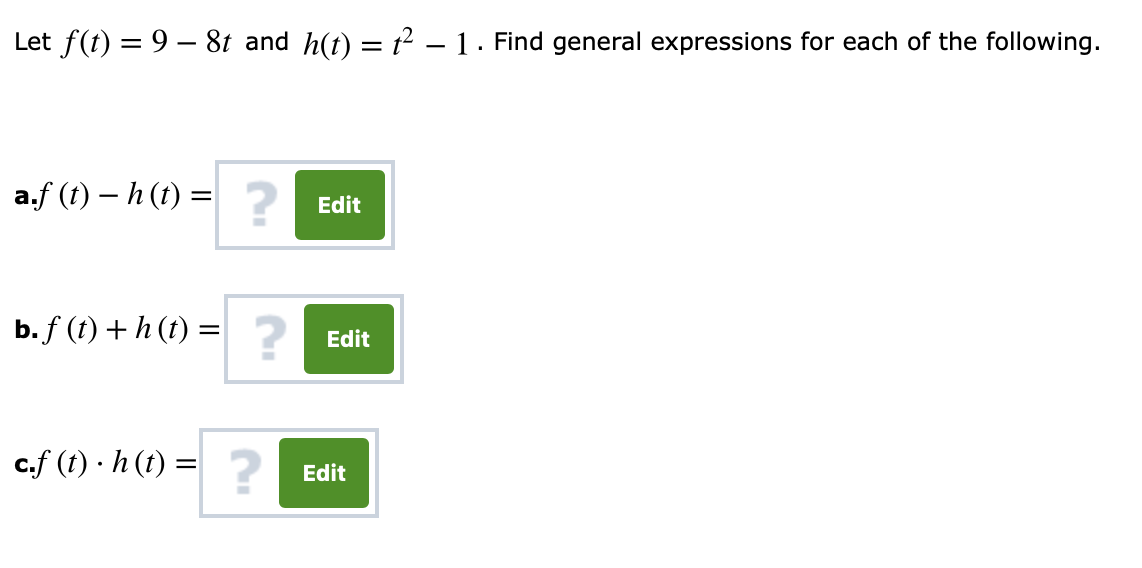# Let f(t) = 9-8t and h(t) = t2-1 . Find general expressions for each of the following. 2 a,f (t)-h (t) = Edit b. f (t) + h (t) = 2 Edit c,f (t) , h (t) = 2 Edit

Questionhelp_outlineImage TranscriptioncloseLet f(t) = 9-8t and h(t) = t2-1 . Find general expressions for each of the following. 2 a,f (t)-h (t) = Edit b. f (t) + h (t) = 2 Edit c,f (t) , h (t) = 2 Edit fullscreen

### Want to see this answer and more?

Experts are waiting 24/7 to provide step-by-step solutions in as fast as 30 minutes!*